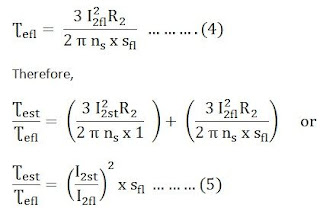# DOL Starter (Direct On Line Starter) Diagram And Working Principle

0
1184

## Types Of Over Load Relays:

### DOL Starter Circuit Diagram:## Relation Between Starting Torque And Full Load Torque Of Induction Motor:

#### rotor copper loss = S x rotor input  (S means slip)#### So we get,#### At full loads = sfl, I2 = I2fl, Ʈe = Ʈefl#### If we neglect no load current, we have#### Equating 6 and 7 equations we get,#### Equating 5 and 8 equations we get,#### Then the current at the starting is given by the equation as shown.#### From equation 9 and 10 we get,